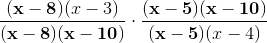# High School Math : Simplifying Rational Expressions

## Example Questions

### Example Question #1 : Rational Expressions

Simplify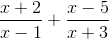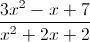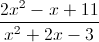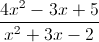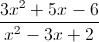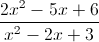Explanation:

This is a more complicated form of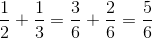Find the least common denominator (LCD) and convert each fraction to the LCD, then add the numerators.  Simplify as needed.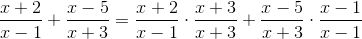which is equivalent to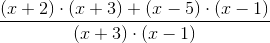Simplify to get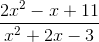### Example Question #3 : Rational Expressions

Divide and simplify the following rational expression: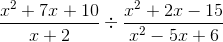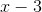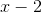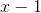Explanation:

Multiply by the reciprocal of the second expression: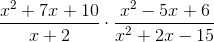Factor the expressions: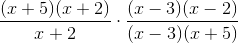Remove common terms: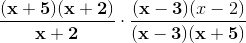### Example Question #4 : Rational Expressions

Add and simplify the following rational expression: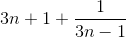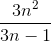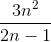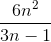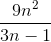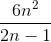Explanation:

Begin by multiplying the left term by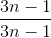: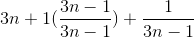Simplify: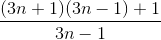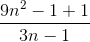### Example Question #5 : Rational Expressions

Simplify the following rational expression: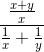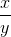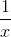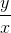Explanation:

Begin by combining the terms in the denominator: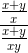Multiply by the reciprocal of the denominator: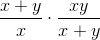Remove like terms: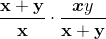### Example Question #6 : Rational Expressions

Simplify the following rational expression: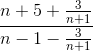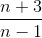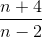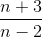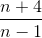Explanation:

Create a common denominator of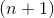in both the numerator and denominator: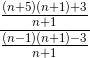Multiply by the reciprocal of the denominator: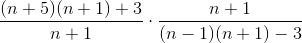Simplify: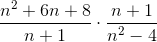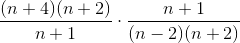Remove common terms: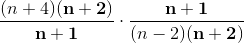### Example Question #7 : Rational Expressions

Multiply and simplify the following rational expression: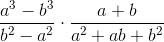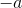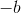Explanation:

Factor the expression: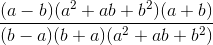Remove like terms: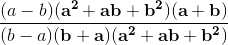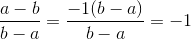### Example Question #8 : Rational Expressions

Divide and simplify the following rational expression: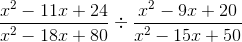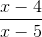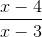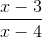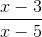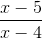Explanation:

Multiply by the inverse of the denominator: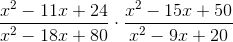Factor: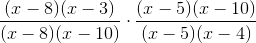Remove like terms: